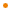# JavaFX: Bringing Rich Experiences To All the Screens Of Your Life

### Overview

This class represents an `Affine` object that rotates coordinates around an anchor point. This operation is equivalent to translating the coordinates so that the anchor point is at the origin (S1), then rotating them about the new origin (S2), and finally translating so that the intermediate origin is restored to the coordinates of the original anchor point (S3).

For example, the matrix representing the returned transform of Rotate { angle: theta pivotX: x pivotY: y pivotZ: z } is :

```              [   cos(theta)    -sin(theta)   0    x-x*cos+y*sin  ]
[   sin(theta)     cos(theta)   0    y-x*sin-y*cos  ]
[      0               0        1          z        ]
```

the code:

``````import javafx.scene.text.*;
import javafx.scene.transform.*;

Text {
transforms: Rotate { angle: 30 }
x: 10 y: 50
font: Font { size: 20 }
content: "This is a test"
}``````

produces:Profile: common

### Script Variable Summary

accessnametypedescription
publicX_AXISPoint3DSpecifies the X-axis as the axis of rotation.

publicY_AXISPoint3DSpecifies the Y-axis as the axis of rotation.

publicZ_AXISPoint3DSpecifies the Z-axis as the axis of rotation.

### Variable Summary

accessnametypedescription
publicangleNumber0.0

Defines the angle of rotation measured in degrees.

publicaxisPoint3DRotate.Z_AXIS

Defines the axis of rotation at the pivot point.

publicpivotXNumber0.0

Defines the X coordinate of the rotation pivot point.

publicpivotYNumber0.0

Defines the Y coordinate of the rotation pivot point.

publicpivotZNumber0.0

Defines the Z coordinate of the rotation pivot point.